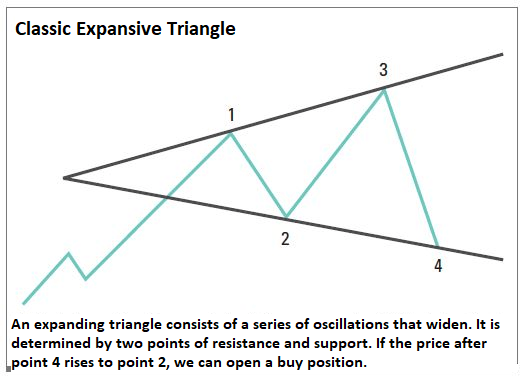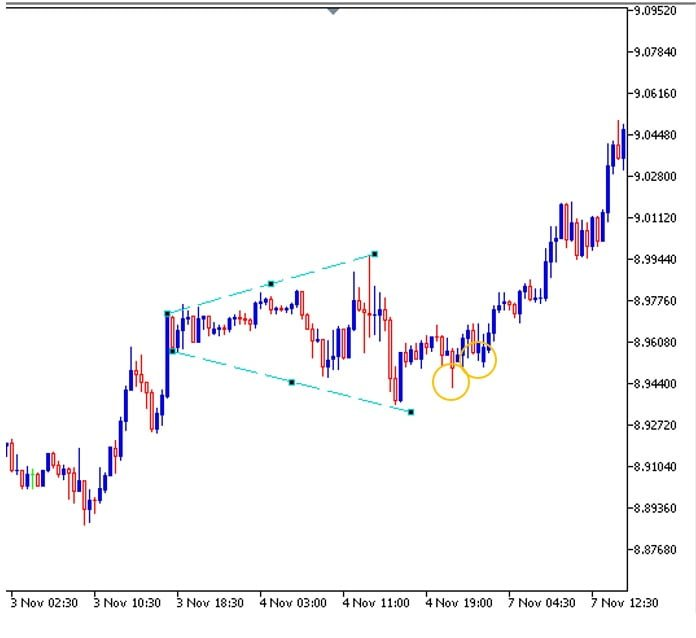# Expanding Triangle – How to trade with this price pattern?

## What is the expanding triangle pattern?

The expanding triangle pattern, also known simply as expansionary formation, is formed during periods of very high market volatility, with many price oscillation and a not very clear trend. With each swing the pattern expands further, forming two opposite trend lines.An expanding triangle consists of a series of swings that widen as price moves. It is determined by two points of resistance and support. If the price after point 4 rises to point 2, we can open a buy position. But if the price after point 3 falls to point 1, we can look to open a sell position.

Since neither the price minimums nor the maximums are equal,  none of the lines in the pattern is horizontal. If we connect the maximum and minimum points we see that both lines have slopes, but they do not start from a vertex. The figure seems to be growing and then forms the expanding triangle.

If we connect the maximum and minimum points we see that both lines have slopes, but they do not start from a vertex. The figure seems to be growing and then forms the expanding triangle.

If we connect the maximum and minimum points we see that both lines have slopes, but they do not start from a vertex contrary to the normal symmetrical triangle. The figure seems to be growing and then forms the expanding triangle.

These formations rarely appear in normal market conditions and if we look at large time frames, we will see that it is not easy to find them, however, in shorter time periods they usually appear constantly.

## How is the expanding triangle formed in the market?

Unlike the other triangles patterns, the expanding triangle does not form in any particular type of trend. It simply appears in some consolidation period after a previous trend, but with sudden price movements and without a clear trend.

As the movements are many and the volatility is high, the difference between the highs and the lows is great. As already said, the trend is not obvious, but if we draw a line at both the lows and the highs, it can be seen that a triangle pattern forms, although it never closes but the trendlines do come close to touching. Because of this situation, it could be said that rather a trapezoid is formed.

## How to trade the expanding triangle when it is formed on the chart?

It is difficult to trade the expansive triangle in trading, first because it appears in both trends, and because there are theories that these patterns can be used for both long and short positions, regardless of the previous trend.

However, it is much more common for the expanding triangle to be used as a continuation pattern, so if the pattern appears in an uptrend then we will use it to take long positions. And, if the triangle appears in a downtrend, we will use it to take short positions.

The expansive triangle does not have a defined strategy either and many traders suggest the use of moving averages or some other indicator, and although it is true that it is recommended to confirm with some other tool, a strategy can also be used solely with the formation of the triangle.

Therefore, if the previous trend is bullish, the long position is taken if the expanding triangle appears and then two lows form at higher prices. The take-profit price would have to be placed at a distance equal to that between the high and low of the triangle. The stop loss would be at the minimum level within the triangle.

In a downtrend, the trader would have to open a short position if after the formation of the triangle pattern, two spikes are formed at lower prices. As with the uptrend, the profit-taking level should be set at a level equal to the distance between highs and lows. The stop loss has to be at the maximum level reached in the triangle.

## Expanding triangle pattern example

Let’s review an example of how to use the expanding triangle in trading:The chart above shows the USD/SEK currency pair with a 30 minute time frame. As we have said, these formations occur when the market is dominated by high volatility and traders cannot decide on a certain direction. In this example, the initial direction of the trend is bullish.

We see that the expansive triangle begins to form at 6.30 pm, after the bullish candlestick. There is a series of lateral movements with minimums and maximums that do not form horizontal lines. After two very marked valleys  that connect with the triangle line, there are two more lows, but at higher levels than the previous ones (indicated in orange circles), then we could consider opening a long position.

If hypothetically the position is taken, the position opening would have to occur around 8.9608. The take ptofit level would then have to be at 9.0252.

We obtain this from the following calculation: Maximum (8.9944) minus the minimum (8.93) = 0.0644. Then, entry price (8.9608) plus the difference (0.0644), this is equal to 8.9608 + 0.0644 = 9.0252. This level was quickly reached on November 7.

The stop loss should be at 8.93, the lowest level in the expansive triangle.

## Conclusion

The strategy we have presented shows a simple way to trade long and short based on the expansive triangle technical pattern. These will allow the trader to avoid false signals due to bullish and bearish market traps.